# How Many x-Intercepts?

## Question

What is the maximum number of zeros (also called x-intercepts) the function    may have? What is the minimum? c, a constant, may be any real number.

## Solution

The maximum number zeros of a polynomial function is equal to the function’s degree. The function  is fourth degree, so it may have up to four zeros. A fourth-degree function may look like this: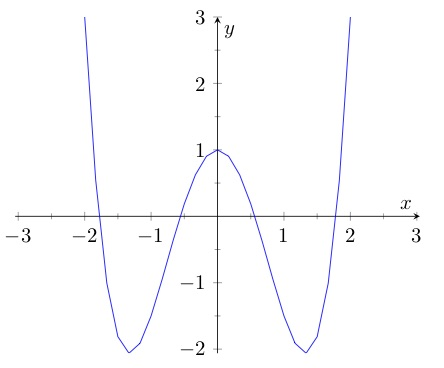Four is the max. Will the function  necessarily have four? Let’s try to narrow down the possibilities.

Try a graph. You can graph this function if you assign a value to c. Let’s try , so that the function is . That graphs up like this:That gives you two x-intercepts. but with a different value of c could this function may have more x-intercepts — or fewer?

At the x-intercepts, the y-value is 0:

That means

That means that for this function, at the zeros, the fourth-degree monomial   equals the linear function . Let’s graph these two pieces separately for  , the value of c that we’ve seen gives two x-intercepts.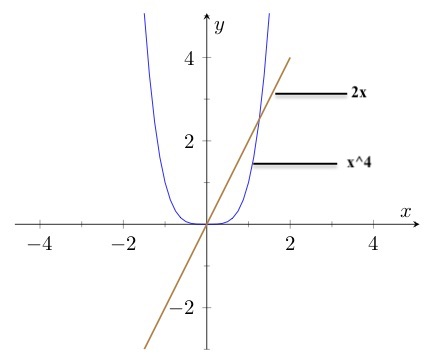These two pieces intersect in two placesone for each of the two zeros. Are any other points of intersection possible? On the left-hand side, the fourth-degree term just keeps going up while the line just keeps going down, so there are no more points of intersection on the left. On the right-hand side to the right of both points of intersection, the fourth-degree term is always steeper than the line and is therefore always greater, so there are no more points of intersection on the right. Remember that each point of intersection on this graph corresponds to a zero on the function , so that means no other zero is possible for the function.

But that doesn’t cover all possibilities, because we considered only one value for c. What if c takes a different value? Then the line slides up or down. If  , the line slides up and there will two points of intersection, one at which x is less than zero and one in which x is greater than zero.

Slide it down just a little from and there are still two points of intersection, this time both positive. Slide it farther and eventually it will not intersect the fourth-degree curve at all.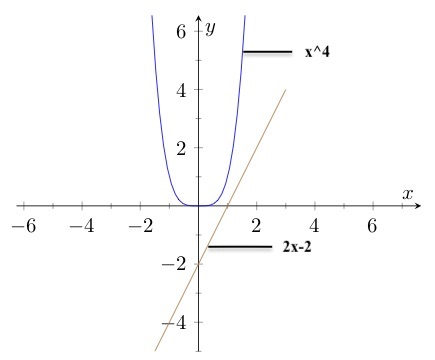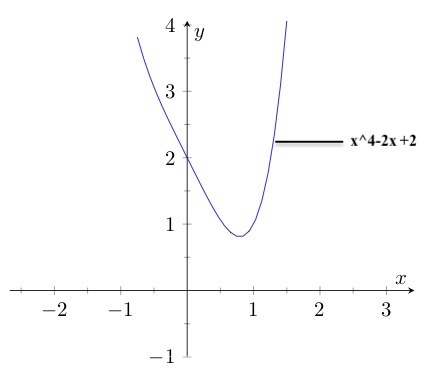For even higher values of c – meaning the line slides farther down on the y-axis – the function has no zeros.

So for the function , the minimum number of x-intercepts is zero and the maximum number is two.

# Synthetic Division

You’re not likely to get a CLEP question that requires you to do synthetic division, but you quite likely could get a question for which you need to divide polynomials. Synthetic division is an algorithm that allows you to divide polynomials faster than you can with regular old polynomial long division (pretty much because you don’t have to keep writing x, x, x . . . .). You can do it even if you never mastered polynomial long division.

Synthetic division works when you divide by a binomial: , where  is some real number.

Here’s how synthetic division works:

Say your problem is . Then  and

• Set up something that looks like this: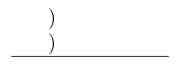• Write in  where  is shown below. To the right of that write in the coefficients of the  terms. The coefficient of  is 1. There is no  or  term, but treat those as if they exist, with coefficients of 0. And the constant term is 8. The setup should look like this:• From the list of coefficients, copy the one farthest to the left – that’s 1 – to directly below itself just below the horizontal line.• Multiply that 1 by the -2 all the way over on the left. Write the result in just above the horizontal line, directly below the next term in the coefficient list (the first 0 in this case).• Add that 0 to the number you just wrote under it, and write the sum below the horizontal line.• Multiply the -2 all the way on the left by the -2 you just wrote under the horizontal line. Write the product under the next entry on the coefficient list, in this case the next 0. Add the number you just wrote to the number above it, and write the sum under the horizontal line. Keep going like that until you are out of numbers in the coefficient line.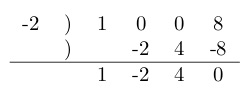The numbers below the line represent the answer. The expression is one degree lower than the one you divided. Since in this case the expression you divided is third degree, the answer is second degree. The first term is the coefficient of , the second term is the coefficient of , the third term is a constant term, and the fourth is the remainder.